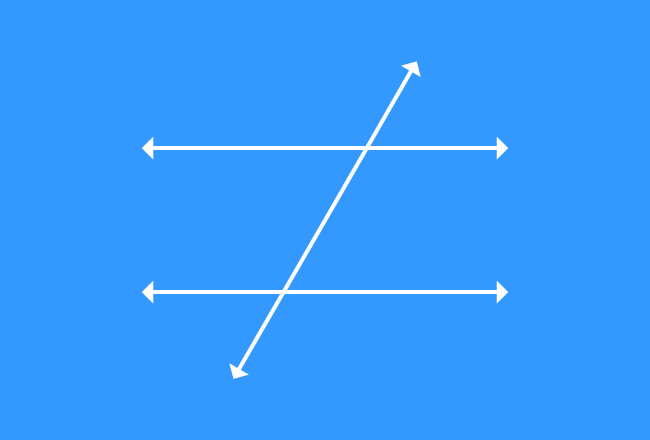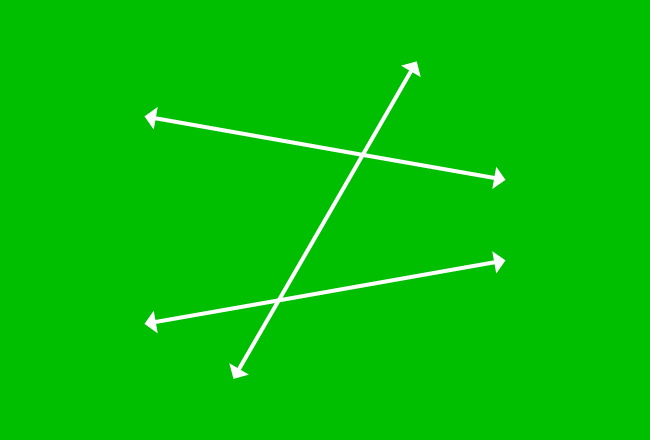# Corresponding Angles formed by Lines and their transversal

Angles are formed geometrically when two or more lines are intersected by their transversal. An angle has similarity in position with one or more angles in this special geometric case and they are known as corresponding angles.

Two straight lines cut by another straight line is a basic case of transversal. In this case, four pairs of corresponding angles are formed geometrically. Now, study the properties of the corresponding angles formed by the intersection of two parallel (or) nonparallel lines and their transversal.

1Learn formation and properties of corresponding angles of transversal of two parallel lines.

2Learn properties and formation of corresponding angles of transversal of two nonparallel lines.

Latest Math Topics
Jun 26, 2023
Jun 23, 2023

###### Math Questions

The math problems with solutions to learn how to solve a problem.

Learn solutions

Practice now

###### Math Videos

The math videos tutorials with visual graphics to learn every concept.

Watch now

###### Subscribe us

Get the latest math updates from the Math Doubts by subscribing us.